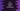# C++ program to convert ASCII to character## C++ program to convert ASCII to character:

ASCII is a character encoding standard for communication. Each character has an ASCII value,for example, the ASCII value of character a is 97, for b is 98 etc.

In some cases, we need to convert an ASCII value to the character it is representing. We can do that in different ways in C++.

In this post, we will learn how to convert ASCII to character in C++.

### By using char():

We can use the char() method to convert any ASCII value to its character equivalent. For example:

``````#include <iostream>

using namespace std;

int main()
{
int asciiValue = 97;
char charValue = char(asciiValue);

cout << "ASCII: " << asciiValue << ", character: " << charValue << endl;

return 0;
}``````

If you run this, it will print the below output:

### Direct conversion:

We can also convert it using the = operator. We don’t have to use char() for that.

``````#include <iostream>

using namespace std;

int main()
{
int asciiValue = 97;
char charValue = asciiValue;

cout << "ASCII: " << asciiValue << ", character: " << charValue << endl;

return 0;
}``````

It will print a similar output as the above program.

``ASCII: 97, character: a``

### How to convert and print the character value in cout:

If you want to convert and print the characters for ASCII values, you can use cout. But, if you directly print any integer value, it will print that number. We can type cast the value to character to print the character equivalent.

For example:

``````#include <iostream>

using namespace std;

int main()
{
int asciiValue = 97;

cout << "ASCII: " << asciiValue << ", character: " << (char)asciiValue << endl;

return 0;
}``````

It will print:

``ASCII: 97, character: a``

Let’s print A to Z using ASCII. We can use a loop and print the Alphabet. The loop will start from 65, which is the ASCII value of A and it will end at 90, which is the ASCII value of Z.

Below program prints A to Z using ASCII values:

``````#include <iostream>

using namespace std;

int main()
{
for (int i = 65; i <= 90; i++)
{
cout << (char)i << ' ';
}

return 0;
}``````

If you run this program, it will print:

``A B C D E F G H I J K L M N O P Q R S T U V W X Y Z``

Here,

• We are running a for loop. We can also use any other loop as well.
• The loop is using integer i to iterate through the loop.
• We are type casting the integer value while printing the values.